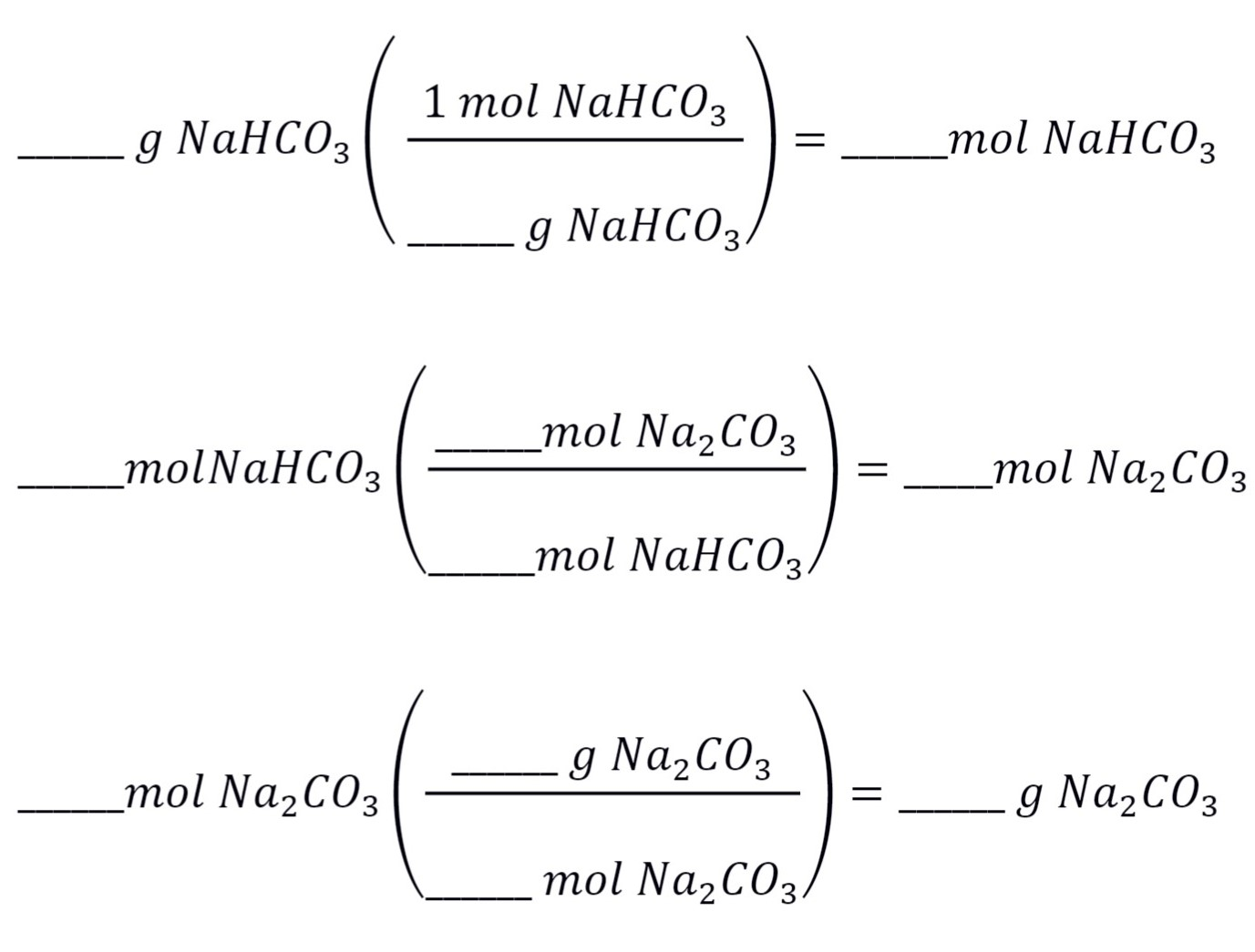# Experiment_604_Thermal_Decomposition_of_Sodium_Bicarbonate_1_2_3

$$\newcommand{\vecs}{\overset { \rightharpoonup} {\mathbf{#1}} }$$ $$\newcommand{\vecd}{\overset{-\!-\!\rightharpoonup}{\vphantom{a}\smash {#1}}}$$$$\newcommand{\id}{\mathrm{id}}$$ $$\newcommand{\Span}{\mathrm{span}}$$ $$\newcommand{\kernel}{\mathrm{null}\,}$$ $$\newcommand{\range}{\mathrm{range}\,}$$ $$\newcommand{\RealPart}{\mathrm{Re}}$$ $$\newcommand{\ImaginaryPart}{\mathrm{Im}}$$ $$\newcommand{\Argument}{\mathrm{Arg}}$$ $$\newcommand{\norm}{\| #1 \|}$$ $$\newcommand{\inner}{\langle #1, #2 \rangle}$$ $$\newcommand{\Span}{\mathrm{span}}$$ $$\newcommand{\id}{\mathrm{id}}$$ $$\newcommand{\Span}{\mathrm{span}}$$ $$\newcommand{\kernel}{\mathrm{null}\,}$$ $$\newcommand{\range}{\mathrm{range}\,}$$ $$\newcommand{\RealPart}{\mathrm{Re}}$$ $$\newcommand{\ImaginaryPart}{\mathrm{Im}}$$ $$\newcommand{\Argument}{\mathrm{Arg}}$$ $$\newcommand{\norm}{\| #1 \|}$$ $$\newcommand{\inner}{\langle #1, #2 \rangle}$$ $$\newcommand{\Span}{\mathrm{span}}$$$$\newcommand{\AA}{\unicode[.8,0]{x212B}}$$

 Student Name Laboratory Date:  Date Report Submitted: ___________________________ Student ID Experiment Number and Title Experiment 604:  Thermal Decomposition of Sodium Bicarbonate

Experiment 604:  Thermal Decomposition of Sodium Bicarbonate

Section 1:  Purpose and Summary

Determine if sodium bicarbonate decomposes by reaction A or reaction B when heated.

 NaHCO3(s)→Na2CO3(s)+H2O(g)+CO2(g) Reaction A Sodium   bicarbonate Sodium carbonate Water vapor Carbon dioxide NaHCO3(s)→Na2O(s)+H2O(g)+CO2(g) Reaction B sodium bicarbonate Sodium oxide Water vapor Carbon dioxide

NaHCO3 is commonly called sodium bicarbonate. When NaHCO3 is heated above 110 °C, H2O and CO2 are produced by a chemical change. After the decomposition reaction is complete, a white solid residue remains. Among all possible reactions, the two possibilities listed above are most likely to explain these observations.

Students are to choose between reactions (A) and (B) on the basis of their laboratory work, which will involve heating a weighed sample of sodium bicarbonate in a crucible and weighing the residue left in the crucible after heating. From the mass of the solid product ("residue"), students should be able to decide if it is Na2CO3 or Na2O.

Section 2:  Safety Precautions and Waste Disposal

Safety Precautions:

Do not attempt to move a hot crucible; allow sufficient time for it to cool.

Use care when handling HCl solutions. If any HCl splashes on your skin or clothing, rinse it off immediately with plenty of water.

Waste Disposal:

The solid waste may be discarded in one of the regular garbage cans.

Section 3: Procedure

Part 1:  Clean and Weigh Crucible

 Obtain a clean, dry crucible that has no cracks. Place the crucible in a clay triangle, on a ring attached to a ring stand. Make certain that the crucible hangs securely in the triangle; ask for help if it seems shaky. Put the lid on the crucible. Heat the crucible and lid for 10 minutes with a high flame, until the bottom of the crucible glows red hot. Allow the crucible to cool in the clay triangle. (This step is to clean out the crucible.) When they have reached room temperature, weigh the crucible and lid. Record the mass in the data table below.

Part 2:  Measure Mass of Residue after Heating

1.  Add sodium bicarbonate to the crucible until it is 3/4 full. Weigh the crucible, sodium bicarbonate, and lid. Record the mass in the data table below.

1.  Obtain the mass of the sodium bicarbonate by subtraction. Show your work below and enter the mass of the sodium bicarbonate in the data table

 Mass of NaHCO3 = Mass of crucible, lid and NaHCO3 - Mass of crucible and lid

1.  Using a medium-sized flame, heat the sample for 15 minutes. Do NOT heat the sample until it is red hot. Cool it in place as before, and then weigh it. Record the mass of the crucible, lid and residue in the data table below.

1.  Calculate the mass of the residue remaining by subtraction. Show your work below and enter the mass of the residue in the data table below.

 Mass of residue = Mass of crucible, lid and residue - Mass of crucible and lid

1.  Heat again, this time for 10 minutes, then cool and weigh as before. Record the mass of the crucible, lid and residue in the data table below.

1.  Calculate the mass of the residue remaining by subtraction. Show your work below and enter the mass of the residue in the data table below.

1.  Heat for 10 minutes for a third time, then cool and weigh as before. Record the mass of the crucible, lid and residue in the data table below.

1.  Calculate the mass of the residue remaining by subtraction. Show your work below and enter the mass of the residue in the data table below.

Part 3: Data Table

 Before heating After first heating After second heating After third heating Mass of crucible, lid & sample (g) Mass of crucible & lid (g) Mass of sample (g)

Part 4: Test for Presence of Carbonate

 Carbonate salts such as Na2CO3 react with acids to form CO2 gas. If the solid fizzes when some HCl solution is added to it, this indicates that the solid is a carbonate or bicarbonate. Fizzing will not be observed if the product is Na2O. Add a few drops of 6 M hydrochloric acid to the solid product in your crucible to double-check your results. Record your observations. Observations:

Section 4: Calculations

Part 1: Balance Chemical Equations

 Before attempting the calculations, balance the chemical equations for reactions A and B below. ____NaHCO3(s)→____Na2CO3(s)+____H2O(g)+____CO2(g) Reaction A Reactants  Na atoms: _______  C atoms: _______  H atoms: _______  O atoms: _______ Products  Na atoms: _______  C atoms: _______  H atoms: _______  O atoms: _______

 ____NaHCO3(s)→____Na2O(s)+____H2O(g)+____CO2(g) Reaction B Reactants  Na atoms: _______  C atoms: _______  H atoms: _______  O atoms: _______ Products  Na atoms: _______  C atoms: _______  H atoms: _______  O atoms: _______

Part 2: Yield Calculations

 Starting with the mass of NaHCO3 used, calculate the theoretical yield of Na2CO3 if reaction A occurred. Show your work below.Theoretical yield of Na2CO3:    __________________________ Starting with the mass of NaHCO3 used, calculate the theoretical yield of Na2O if reaction A occurred. Show your work below. Theoretical yield of Na2O:    __________________________ Calculate the percent yield of Na2CO3 assuming reaction A occurred. Show you work below.      % _____ yield= $$\frac{\text {actual yield}}{\text {theoretical yield}}$$ ×100 Percent yield of Na2CO3:    __________________________

 Post Lab Questions:    Compare the observed mass of residue after heating to the predicted masses of Na2CO3 and Na2O. Which reaction actually occurred and which product was formed? Consider the percent yield calculated for Na2CO3 and the percent yield calculated for Na2O. Do these numbers support your conclusion about which reaction is really occurring? How? Did your observations during Part 4: Test for Presence of Carbonate agree with your conclusions based on the weight data? Explain?

Notes:

Experiment_604_Thermal_Decomposition_of_Sodium_Bicarbonate_1_2_3 is shared under a CC BY license and was authored, remixed, and/or curated by LibreTexts.